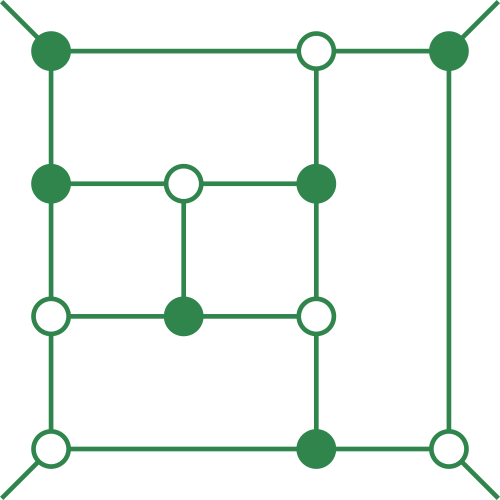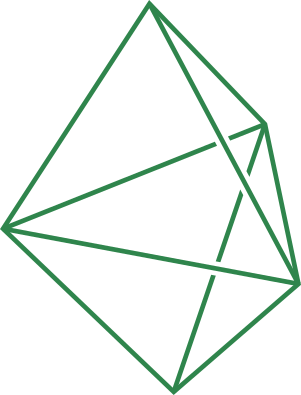Abstracts

 Niklas Beisert Yangian Symmetry of Planar N=4 SYM (slides) This talk is about integrability of Planar N=4 super Yang-Mills theory. We present a concrete notion of the corresponding Yangian symmetry in this model, show that it holds true, and discuss the (perturbative) implications for correlation functions and Wilson loops. Jacob Bourjaily Grassmannian Geometry Beyond the Planar Limit Lance Dixon The Steinmann-assisted bootstrap at five loops (slides) The Steinmann relations forbid double discontinuities in overlapping channels. They powerfully constrain six-gluon scattering in planar N=4 super-Yang-Mills theory. We also assume that the answer is a hexagon function, i.e. an iterated integral with a nine letter symbol and no unphysical discontinuities. These twin constraints lead to unique answers through five loops for the MHV and NMHV amplitudes with minimal additional assumptions. In addition, the entire Steinmann-satisfying hexagon function space, modulo certain restrictions on zeta values, appears to be required to describe all the derivatives of high-loop amplitudes. In other words, the function space is not too big, and not too small, but just right! Daniele Galloni The Geometry of Non-Planar On-Shell Diagrams (slides) There is an increasing amount of evidence suggesting that non-planar amplitudes may be expressed using non-planar on-shell diagrams. The structure and diversity of such diagrams is nearly completely unexplored. After developing a method for constructing the singularity structure associated to non-planar on-shell diagrams, I will give a guided tour of the full wealth of structures in G(3,6). Paul Heslop Amplitudes and Correlators to Ten Loops Using Simple, Graphical Bootstraps (slides) We introduce two new graphical-level relations among possible contributions to the four-point correlation function and scattering amplitude in planar, maximally supersymmetric Yang-Mills theory. When combined with the rung rule, these prove powerful enough to fully determine the correlator and amplitude through ten loops. This then also directly yields the full five-point amplitude to eight loops and the parity even part to nine loops. We derive these rules, illustrate their applications, and compare their relative strengths for fixing coefficients. We survey some of the features of the previously unknown nine and ten loop expressions. Finally we will discuss the translation of these and other expressions into a "correlahedron" space. Arthur Lipstein On-shell Diagrams for N=8 Supergravity Amplitudes (slides) Standard Feynman diagram techniques often obscure the underlying simplicity of on-shell scattering amplitudes. This difficulty can be overcome by working with on-shell diagrams, which do not contain virtual particles. In this talk, I will describe tree-level recursion relations for N=8 supergravity amplitudes using a generalisation of the on-shell diagrams developed for planar N=4 super-Yang-Mills, which imply new identities for non-planar diagrams as well as interesting relations between the N=4 and N=8 theories. If time permits, I will also speculate on the extension of this approach to loop-level. This talk is primarily based on 1604.03046, in collaboration with Paul Heslop. Tomasz Łukowski Towards the Amplituhedron volume Lionel Mason Ambitwistor strings for trees and loops (slides) This talk will give an introduction to, and overview of aspects of ambitwistor strings, in particular their geometric origins, extending the models for different matter fields, and formulae for loop amplitudes. Jan Plefka The near collinear limit of gluon amplitudes and novel relations to Einstein-Yang-Mills amplitudes (slides) The structure of tree-level scattering amplitudes for collinear massless bosons is studied beyond their leading splitting function behavior. These near-collinear limits at sub-leading order are best studied using the Cachazo-He-Yuan (CHY) formulation of the S-matrix based on the scattering equations. We compute the collinear limits for gluons, gravitons and scalars. It is shown that the CHY integrand for an n-particle gluon scattering amplitude in the collinear limit at sub-leading order is expressed as a convolution of an (n-1)-particle gluon integrand and a collinear kernel integrand, which is universal. Our representation is shown to obey recently proposed amplitude relations in which the collinear gluons of same helicity are replaced by a single graviton. Moreover, we present new relations for scattering amplitudes of color ordered gluons and gravitons in Einstein-Yang-Mills theory. Tree-level amplitudes of arbitrary multiplicities and polarizations involving up to three gravitons and up to two color traces are reduced to partial amplitudes of pure Yang-Mills theory. Matthias Staudacher Yangian Invariants, Unitary Matrix Models, and Scattering Amplitudes Gabriele Travaglini Amplitudes and form factors from N=4 super Yang-Mills to QCD (slides) Jaroslav Trnka Evidence for Non-planar Amplituhedron (slides) The Amplituhedron provides a geometric formulation of planar integrand in N=4 SYM theory. Beyond the planar limit this construction does not naively exist anymore as hidden symmetries are absent and there are no momentum twistors which are crucial for the Amplituhedron definition. In this talk I will show that when translating the implications of the Amplituhedron construction to the momentum space the non-planar sector satisfies the same constraints as the planar one. This gives an evidence for the hidden geometric structure in the complete N=4 SYM theory. I will also comment on challenges when pushing this program further to N=8 SUGRA.